library: libGeom#include "TGeoTube.h"

# TGeoTubeSeg

class description - source file - inheritance tree (.pdf)

## class TGeoTubeSeg : public TGeoTube

Inheritance Chart:
 TObject
<-
TNamed
<-
TGeoShape
<-
TGeoBBox
<-
TGeoTube
<-
TGeoTubeSeg
<-
 TGeoCtub
```
public:
TGeoTubeSeg()
TGeoTubeSeg(Double_t rmin, Double_t rmax, Double_t dz, Double_t phi1, Double_t phi2)
TGeoTubeSeg(const char* name, Double_t rmin, Double_t rmax, Double_t dz, Double_t phi1, Double_t phi2)
TGeoTubeSeg(Double_t* params)
TGeoTubeSeg(const TGeoTubeSeg&)
virtual ~TGeoTubeSeg()
static TClass* Class()
virtual void ComputeBBox()
virtual void ComputeNormal(Double_t* point, Double_t* dir, Double_t* norm)
static void ComputeNormalS(Double_t* point, Double_t* dir, Double_t* norm, Double_t rmin, Double_t rmax, Double_t dz, Double_t c1, Double_t s1, Double_t c2, Double_t s2)
virtual Bool_t Contains(Double_t* point) const
virtual Int_t DistancetoPrimitive(Int_t px, Int_t py)
virtual Double_t DistFromInside(Double_t* point, Double_t* dir, Int_t iact = 1, Double_t step = TGeoShape::Big(), Double_t* safe = 0) const
static Double_t DistFromInsideS(Double_t* point, Double_t* dir, Double_t rmin, Double_t rmax, Double_t dz, Double_t c1, Double_t s1, Double_t c2, Double_t s2, Double_t cm, Double_t sm, Double_t cdfi)
virtual Double_t DistFromOutside(Double_t* point, Double_t* dir, Int_t iact = 1, Double_t step = TGeoShape::Big(), Double_t* safe = 0) const
static Double_t DistFromOutsideS(Double_t* point, Double_t* dir, Double_t rmin, Double_t rmax, Double_t dz, Double_t c1, Double_t s1, Double_t c2, Double_t s2, Double_t cm, Double_t sm, Double_t cdfi)
virtual TGeoVolume* Divide(TGeoVolume* voldiv, const char* divname, Int_t iaxis, Int_t ndiv, Double_t start, Double_t step)
virtual Double_t GetAxisRange(Int_t iaxis, Double_t& xlo, Double_t& xhi) const
virtual void GetBoundingCylinder(Double_t* param) const
virtual const TBuffer3D& GetBuffer3D(Int_t reqSections, Bool_t localFrame) const
virtual Int_t GetByteCount() const
virtual TGeoShape* GetMakeRuntimeShape(TGeoShape* mother, TGeoMatrix* mat) const
virtual Int_t GetNmeshVertices() const
Double_t GetPhi1() const
Double_t GetPhi2() const
virtual void InspectShape() const
virtual TClass* IsA() const
virtual TBuffer3D* MakeBuffer3D() const
TGeoTubeSeg& operator=(const TGeoTubeSeg&)
virtual Double_t Safety(Double_t* point, Bool_t in = kTRUE) const
static Double_t SafetyS(Double_t* point, Bool_t in, Double_t rmin, Double_t rmax, Double_t dz, Double_t phi1, Double_t phi2, Int_t skipz = 0)
virtual void SavePrimitive(ofstream& out, Option_t* option)
virtual void SetDimensions(Double_t* param)
virtual void SetPoints(Double_t* points) const
virtual void SetPoints(Float_t* points) const
virtual void SetSegsAndPols(TBuffer3D& buff) const
void SetTubsDimensions(Double_t rmin, Double_t rmax, Double_t dz, Double_t phi1, Double_t phi2)
virtual void ShowMembers(TMemberInspector& insp, char* parent)
virtual void Sizeof3D() const
virtual void Streamer(TBuffer& b)
void StreamerNVirtual(TBuffer& b)
```

### Data Members

```
protected:
Double_t fPhi1  first phi limit
Double_t fPhi2  second phi limit
```

## Class Description

``` TGeoTube - cylindrical tube class. It takes 3 parameters :

_____________________________________________________________________________
```
/*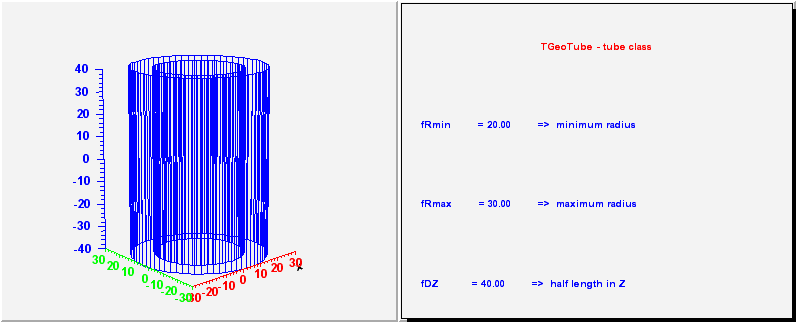*/

/*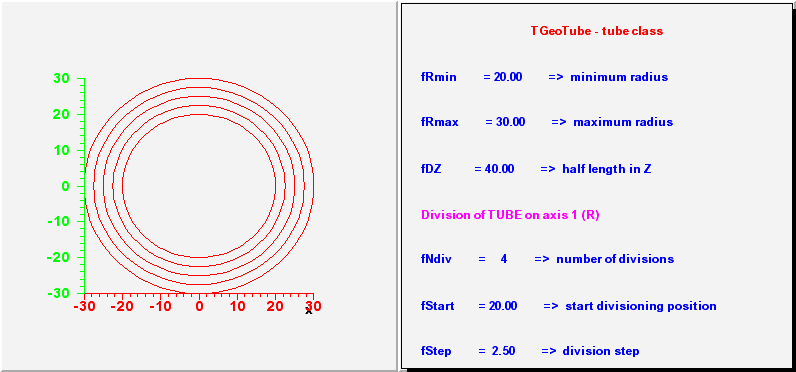*/

/*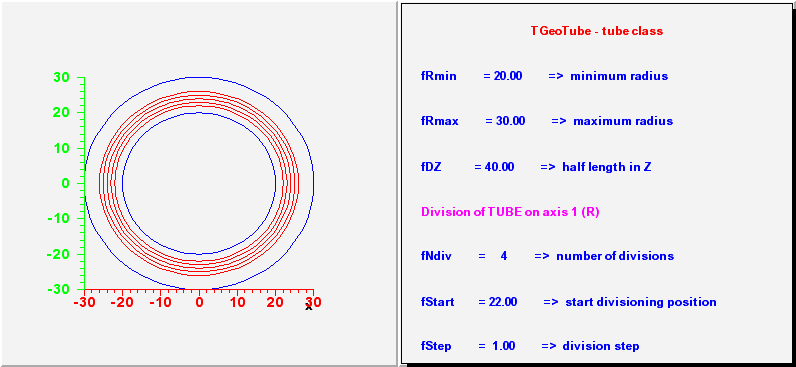*/

/*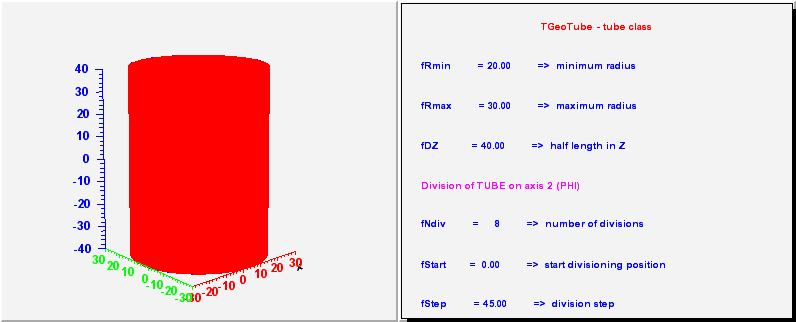*/

/*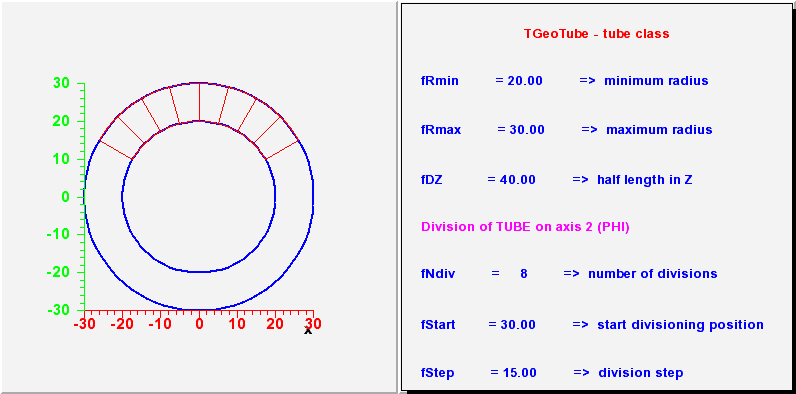*/

/*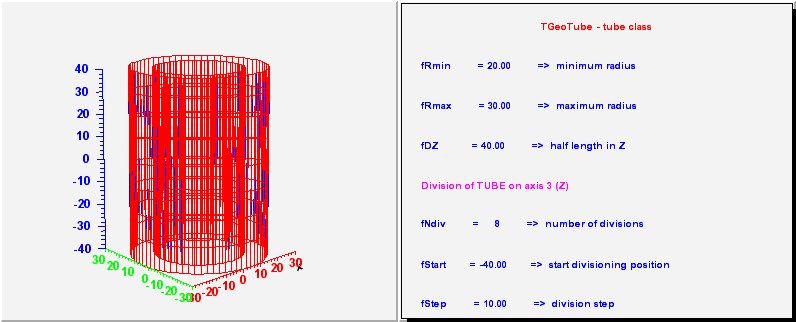*/

/**/

```_____________________________________________________________________________
TGeoTubeSeg - a phi segment of a tube. Has 5 parameters :
- the same 3 as a tube;
- first phi limit (in degrees)
- second phi limit

_____________________________________________________________________________
```
/**/

/**/

/**/

/**/

```_____________________________________________________________________________
TGeoCtub - a tube segment cut with 2 planes. Has 11 parameters :
- the same 5 as a tube segment;
- x, y, z components of the normal to the -dZ cut plane in
point (0, 0, -dZ);
- x, y, z components of the normal to the +dZ cut plane in
point (0, 0, dZ);

_____________________________________________________________________________
```
/**/

TGeoTubeSeg()
``` Default constructor
```

TGeoTubeSeg(Double_t rmin, Double_t rmax, Double_t dz, Double_t phi1, Double_t phi2) :TGeoTube(rmin, rmax, dz)
``` Default constructor specifying minimum and maximum radius
```

TGeoTubeSeg(const char *name, Double_t rmin, Double_t rmax, Double_t dz, Double_t phi1, Double_t phi2) :TGeoTube(name, rmin, rmax, dz)
``` Default constructor specifying minimum and maximum radius
```

TGeoTubeSeg(Double_t *param) :TGeoTube(0, 0, 0)
``` Default constructor specifying minimum and maximum radius
param = Rmin
param = Rmax
param = dz
param = phi1
param = phi2
```

~TGeoTubeSeg()
``` destructor
```

void ComputeBBox()
``` compute bounding box of the tube segment
```

void ComputeNormal(Double_t *point, Double_t *dir, Double_t *norm)
``` Compute normal to closest surface from POINT.
```

void ComputeNormalS(Double_t *point, Double_t *dir, Double_t *norm, Double_t rmin, Double_t rmax, Double_t /*dz*/, Double_t c1, Double_t s1, Double_t c2, Double_t s2)
``` Compute normal to closest surface from POINT.
```

Bool_t Contains(Double_t *point) const
``` test if point is inside this tube segment
first check if point is inside the tube
```

Int_t DistancetoPrimitive(Int_t px, Int_t py)
``` compute closest distance from point px,py to each corner
```

Double_t DistFromInsideS(Double_t *point, Double_t *dir, Double_t rmin, Double_t rmax, Double_t dz, Double_t c1, Double_t s1, Double_t c2, Double_t s2, Double_t cm, Double_t sm, Double_t cdfi)
``` Compute distance from inside point to surface of the tube segment (static)
Boundary safe algorithm.
Do Z
```

Double_t DistFromInside(Double_t *point, Double_t *dir, Int_t iact, Double_t step, Double_t *safe) const
``` Compute distance from inside point to surface of the tube segment
Boundary safe algorithm.
```

Double_t DistFromOutsideS(Double_t *point, Double_t *dir, Double_t rmin, Double_t rmax, Double_t dz, Double_t c1, Double_t s1, Double_t c2, Double_t s2, Double_t cm, Double_t sm, Double_t cdfi)
``` Static method to compute distance to arbitrary tube segment from outside point
Boundary safe algorithm.
```

Double_t DistFromOutside(Double_t *point, Double_t *dir, Int_t iact, Double_t step, Double_t *safe) const
``` compute distance from outside point to surface of the tube segment
fist localize point w.r.t tube
```

TGeoVolume* Divide(TGeoVolume *voldiv, const char *divname, Int_t iaxis, Int_t ndiv, Double_t start, Double_t step)
```--- Divide this tube segment shape belonging to volume "voldiv" into ndiv volumes
called divname, from start position with the given step. Returns pointer
to created division cell volume in case of Z divisions. For radialdivision
creates all volumes with different shapes and returns pointer to volume that
was divided. In case a wrong division axis is supplied, returns pointer to
volume that was divided.
```

Double_t GetAxisRange(Int_t iaxis, Double_t &xlo, Double_t &xhi) const
``` Get range of shape for a given axis.
```

void GetBoundingCylinder(Double_t *param) const
```--- Fill vector param with the bounding cylinder parameters. The order
is the following : Rmin, Rmax, Phi1, Phi2
```

TGeoShape* GetMakeRuntimeShape(TGeoShape *mother, TGeoMatrix * /*mat*/) const
``` in case shape has some negative parameters, these has to be computed
in order to fit the mother
```

void InspectShape() const
``` print shape parameters
```

TBuffer3D* MakeBuffer3D() const
``` Creates a TBuffer3D describing *this* shape.
Coordinates are in local reference frame.
```

void SetSegsAndPols(TBuffer3D &buff) const
``` Fill TBuffer3D structure for segments and polygons.
```

Double_t Safety(Double_t *point, Bool_t in) const
``` computes the closest distance from given point to this shape, according
to option. The matching point on the shape is stored in spoint.
```

Double_t SafetyS(Double_t *point, Bool_t in, Double_t rmin, Double_t rmax, Double_t dz, Double_t phi1, Double_t phi2, Int_t skipz)
``` Static method to compute the closest distance from given point to this shape.
```

void SavePrimitive(ofstream &out, Option_t * /*option*/)
``` Save a primitive as a C++ statement(s) on output stream "out".
```

void SetTubsDimensions(Double_t rmin, Double_t rmax, Double_t dz, Double_t phi1, Double_t phi2)

void SetDimensions(Double_t *param)

void SetPoints(Double_t *points) const
``` create sphere mesh points
```

void SetPoints(Float_t *points) const
``` create sphere mesh points
```

Int_t GetNmeshVertices() const
``` Return number of vertices of the mesh representation
```

void Sizeof3D() const
```/// fill size of this 3-D object
/    TVirtualGeoPainter *painter = gGeoManager->GetGeomPainter();
/    if (!painter) return;

/    Int_t n = gGeoManager->GetNsegments()+1;
/    Int_t numPoints = n*4;
/    Int_t numSegs   = n*8;
/    Int_t numPolys  = n*4-2;

```

const TBuffer3D& GetBuffer3D(Int_t reqSections, Bool_t localFrame) const

### Inline Functions

```               Int_t GetByteCount() const
Double_t GetPhi1() const
Double_t GetPhi2() const
TClass* Class()
TClass* IsA() const
void ShowMembers(TMemberInspector& insp, char* parent)
void Streamer(TBuffer& b)
void StreamerNVirtual(TBuffer& b)
TGeoTubeSeg TGeoTubeSeg(const TGeoTubeSeg&)
TGeoTubeSeg& operator=(const TGeoTubeSeg&)
```

Author: Andrei Gheata 24/10/01
Last update: root/geom:\$Name: \$:\$Id: TGeoTube.cxx,v 1.64 2005/08/30 09:58:41 brun Exp \$